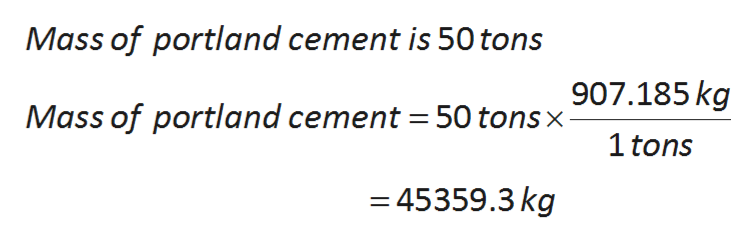# Portland cement contains 61 % calcium oxide. Calculate the mass of calcium oxide and the mass of the rest of the components in kg if the mass of a Portland cement sample is 50 tons.

Question
82 views

Portland cement contains 61 % calcium oxide. Calculate the mass of calcium oxide and the mass of the rest of the components in kg if the mass of a Portland cement sample is 50 tons.

check_circle

Step 1

One ton is equal to 907.185 kgs.

The conversion factor is  (1ton/907.185 kgs )  or  (907.185 kgs/1ton).

To calculation of mass of portland cement as follows:help_outlineImage TranscriptioncloseMass of portland cement is 50 tons 907.185kg Mass of portland cement = 50 tons x 1 tons =45359.3 kg fullscreen
Step 2

Percentage of calcium oxide in portland cement is 61 %.
Mass of portland cement is 45359.3 kg.

Calculate the mass of CaO is as follows:

Step 3

Calculate the mass of the rest of the components is as fo...

### Want to see the full answer?

See Solution

#### Want to see this answer and more?

Solutions are written by subject experts who are available 24/7. Questions are typically answered within 1 hour.*

See Solution
*Response times may vary by subject and question.
Tagged in

### Chemistry